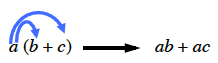Home > CC2MN > Chapter cc25 > Lesson cc25.3.4 > Problem5-140

5-140.

Use the Distributive Property to simplify the following expressions. Homework Help ✎

The Distributive Property involves multiplying the number outside the parentheses into each individual value inside the parentheses and then combining the terms.1. $4(x+2)$

1. $-5(9+x)$

$-45-5x$

Be sure you know how to get this answer.

1. $7(x-3)$